# Multi-Digit Multiplication

Students are introduced to multi-digit multiplication and its applications, including multiplicative comparison.

Math

Unit 2

## Unit Summary

In Unit 2, 4th grade students multiply up to four-digit numbers by one-digit numbers, relying on their understanding of place value and properties of operations, as well as visual models like an area model, to solve.

As a foundation for their multi-year work with multiplication and division, students in 2nd grade learned to partition a rectangle into rows and columns and write a repeated addition sentence to determine the total. They also skip-counted by 5s, 10s, and 100s. Then, in 3rd grade, students developed a conceptual understanding of multiplication and division in relation to equal groups, arrays, and area. They developed a variety of strategies to build toward fluency with multiplication and division within 100 and applied that knowledge to the context of one- and two-step problems using the four operations.

To begin the unit, students extend their understanding of multiplication situations that they learned in 3rd grade to include multiplicative comparison using the words “times as many.” This helps acquaint students with these types of problems before solving them with larger quantities later in the unit. It also gives students time to refresh their multiplication and division facts from 3rd grade, since the problems in Topic A are limited to those that involve multiplication and division within 100. Then, students move into two-digit by one-digit, three-digit by one-digit, four-digit by one-digit, and two-digit by two-digit multiplication, using the area model, partial products, and finally the standard algorithm, making connections between all methods as they go. The use of the area model serves to help students conceptually understand multiplication and as a connection to their work with area and perimeter (4.MD.3), a supporting cluster standard. Finally, with a full understanding of all multiplication cases, they then apply their new multiplication skills to solve multi-step word problems using multiplication, addition, and subtraction, including cases involving multiplicative comparison (4.NBT.5, 4.OA.3, 4.MD.3), allowing for many opportunities to connect content across multiple domains.

This unit affords lots of opportunities to deepen students’ mathematical practices. For example, “when students decompose numbers into sums of multiples of base-ten units to multiply them, they are seeing and making use of structure (MP.7). Further, “by reasoning repeatedly (MP.8) about the connection between math drawings and written numerical work, students can come to see multiplication and division algorithms as abbreviations or summaries of their reasoning about quantities” (NBT Progression, p. 14). Lastly, as students solve multi-step word problems involving addition, subtraction, and multiplication, they are modeling with mathematics (MP.4).

Students’ work in this unit will prepare them for fluency with the multiplication algorithm in 5th grade (5.NBT.5). Students also learn about new applications of multiplication in future grades, including scaling quantities up and down in 5th grade (5.NF.5), all the way up to rates and slopes in the middle grades (6.RP, 7.RP). Every subsequent grade level depends on the understanding of multiplication and its algorithm, making this unit an important one for students in 4th grade.

Pacing: 21 instructional days (18 lessons, 2 flex days, 1 assessment day)

Fishtank Plus for Math

Unlock features to optimize your prep time, plan engaging lessons, and monitor student progress.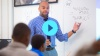## Assessment

The following assessments accompany Unit 2.

### Pre-Unit

Have students complete the Pre-Unit Assessment and Pre-Unit Student Self-Assessment before starting the unit. Use the Pre-Unit Assessment Analysis Guide to identify gaps in foundational understanding and map out a plan for learning acceleration throughout the unit.

### Mid-Unit

Have students complete the Mid-Unit Assessment after lesson 10.

### Post-Unit

Use the resources below to assess student understanding of the unit content and action plan for future units.

Expanded Assessment Package

Use student data to drive your planning with an expanded suite of unit assessments to help gauge students’ facility with foundational skills and concepts, as well as their progress with unit content.

## Unit Prep

### Intellectual Prep

Unit Launch

Prepare to teach this unit by immersing yourself in the standards, big ideas, and connections to prior and future content. Unit Launches include a series of short videos, targeted readings, and opportunities for action planning.#### Intellectual Prep for All Units

• Read and annotate “Unit Summary” and “Essential Understandings” portion of the unit plan.
• Do all the Target Tasks and annotate them with the “Unit Summary” and “Essential Understandings” in mind.
• Take the Post-Unit Assessment.

#### Unit-Specific Intellectual Prep

• Read pp. 14–15 in Progressions for the Common Core State Standards in Mathematics Number and Operations in Base Ten, K-5 (starting at the section titled “Use place value understanding and properties of operations to perform multi-digit arithmetic”).
• Read the document “Situation Types for Operations in Word Problems” for multiplication and division. Identify the word problem types of any applicable assessment questions.
• Read the following table that includes methods used throughout the unit.
 Models for up to 4-digit by 1-digit multiplication Written numerical work Base ten block array (remedial - can be concrete or pictorial)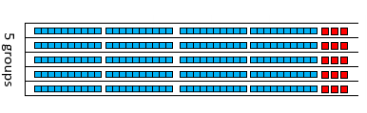Horizontally written partial products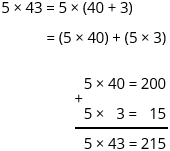Graph paper array (remedial)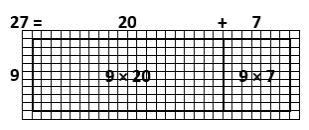Partial product algorithm with decreasing partial products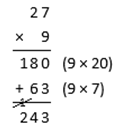Area model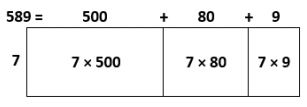Partial product algorithm with increasing partial products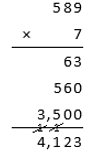Standard algorithm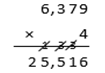Models for up to 2-digit by 2-digit Multiplication Written Numerical Work Base ten block array (remedial - can be concrete or pictorial)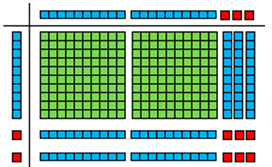Horizontally written partial products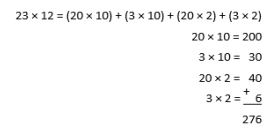Graph paper array (remedial)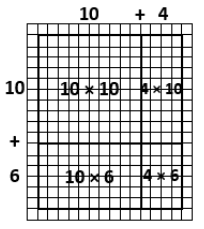Partial product algorithm with decreasing partial products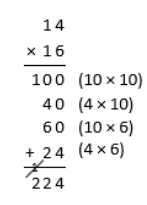Area model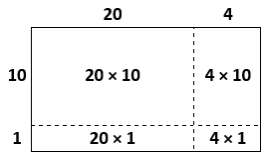Partial product algorithm with increasing partial products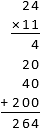Standard algorithm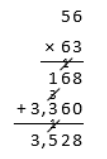### Essential Understandings

• In an additive comparison, the underlying question is what amount would be added to one quantity in order to result in the other. In a multiplicative comparison, the underlying question is what factor would multiply one quantity in order to result in the other.
• One component of understanding general methods for multiplication is understanding how to compute products of one-digit numbers and multiples of 10, 100, and 1,000. Another part of understanding general base-ten methods for multi-digit multiplication is understanding the role played by the distributive property.
• Rounding numbers can help one to determine whether an answer is reasonable based on whether the estimate is close to the computed answer or not.
• Making sense of problems and persevering in solving them is an important practice when solving word problems. Key words do not always indicate the correct operation.

### Vocabulary

partial product

To see all the vocabulary for Unit 2, view our 4th Grade Vocabulary Glossary.

### Materials

• Optional: Base ten blocks (Maximum of 18 thousands, 18 hundreds, 18 tens, 18 ones per student or small group) — Students might not need these depending on their reliance on concrete materials. See Lesson 4 Anchor Task 1 Notes for more information.

## Unit Practice

Word Problems and Fluency Activities

Access daily word problem practice and our content-aligned fluency activities created to help students strengthen their application and fluency skills.## Lesson Map

Topic A: Multiplicative Comparison

Topic B: Multiplication of up to Four-Digit Whole Numbers by One-Digit Whole Numbers

Topic C: Multiplication of Two-Digit Whole Numbers by Two-Digit Whole Numbers

Topic D: Multi-Step Word Problems

## Common Core Standards

Key

Major Cluster

Supporting Cluster

### Core Standards

#### Measurement and Data

• 4.MD.A.3 — Apply the area and perimeter formulas for rectangles in real world and mathematical problems. For example, find the width of a rectangular room given the area of the flooring and the length, by viewing the area formula as a multiplication equation with an unknown factor.

#### Number and Operations in Base Ten

• 4.NBT.B.5 — Multiply a whole number of up to four digits by a one-digit whole number, and multiply two two-digit numbers, using strategies based on place value and the properties of operations. Illustrate and explain the calculation by using equations, rectangular arrays, and/or area models.

#### Operations and Algebraic Thinking

• 4.OA.A.1 — Interpret a multiplication equation as a comparison, e.g., interpret 35 = 5 × 7 as a statement that 35 is 5 times as many as 7 and 7 times as many as 5. Represent verbal statements of multiplicative comparisons as multiplication equations.
• 4.OA.A.2 — Multiply or divide to solve word problems involving multiplicative comparison, e.g., by using drawings and equations with a symbol for the unknown number to represent the problem, distinguishing multiplicative comparison from additive comparison.
• 4.OA.A.3 — Solve multistep word problems posed with whole numbers and having whole-number answers using the four operations, including problems in which remainders must be interpreted. Represent these problems using equations with a letter standing for the unknown quantity. Assess the reasonableness of answers using mental computation and estimation strategies including rounding.

• 3.MD.C.7.B
• 3.MD.C.7.C
• 3.MD.D.8

• 3.NBT.A.3
• 4.NBT.A.1
• 4.NBT.A.2
• 4.NBT.A.3
• 4.NBT.B.4

• 3.OA.A.1
• 3.OA.A.2
• 3.OA.A.3
• 3.OA.A.4
• 3.OA.B.5
• 3.OA.B.6
• 3.OA.C.7
• 3.OA.D.8

• 4.NBT.B.6
• 5.NBT.B.5

• 4.NF.A.1
• 4.NF.B.4
• 5.NF.B.3
• 5.NF.B.4
• 5.NF.B.5
• 5.NF.B.5.A
• 5.NF.B.5.B
• 5.NF.B.6

### Standards for Mathematical Practice

• CCSS.MATH.PRACTICE.MP1 — Make sense of problems and persevere in solving them.

• CCSS.MATH.PRACTICE.MP2 — Reason abstractly and quantitatively.

• CCSS.MATH.PRACTICE.MP3 — Construct viable arguments and critique the reasoning of others.

• CCSS.MATH.PRACTICE.MP4 — Model with mathematics.

• CCSS.MATH.PRACTICE.MP5 — Use appropriate tools strategically.

• CCSS.MATH.PRACTICE.MP6 — Attend to precision.

• CCSS.MATH.PRACTICE.MP7 — Look for and make use of structure.

• CCSS.MATH.PRACTICE.MP8 — Look for and express regularity in repeated reasoning.

Unit 1

Place Value, Rounding, Addition, and Subtraction

Unit 3

Multi-Digit Division

## Request a Demo

See all of the features of Fishtank in action and begin the conversation about adoption.

Yes

No

### Any other information you would like to provide about your school?